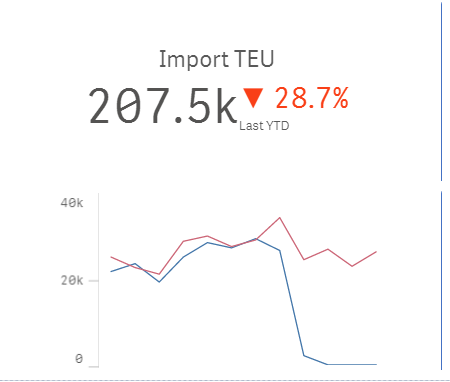# New to Qlik Sense

If you’re new to Qlik Sense, start with this Discussion Board and get up-to-speed quickly.

Announcements
QlikWorld online is next week! REGISTER NOW
cancel
Showing results for
Did you mean:Creator

## Set analysis - show only the current year FOR THE GRAPH doesnt work

Thanks to

Now, if I want to show the same calculation but as a trendline showing MoM trend, the below formula works but it changes as soon as a person made a selection. How could I make the graph static?

count({1}{1<[TimeStamp.Calendar.Year] = {"\$(=year(today()))"},type={'move'},vessel_crane-={"=len(trim(vessel_crane))=0"},
visit-={"=len(trim(visit))=0"},CRANE-={"=len(trim(CRANE))=0"}>} container)
/sum({1}distinct {1<[TimeStamp.Calendar.Year] = {"\$(=year(today()))"},vessel_crane-={"=len(trim(vessel_crane))=0"},
visit-={"=len(trim(visit))=0"},CRANE-={"=len(trim(CRANE))=0"}>}aggr((if(((t_max - t_min)*24) <0,0,(t_max - t_min)*24)), vessel_crane))

1 Solution

Accepted SolutionsPartner

Hi, so in the end it was the IF statement inside of the Aggr.

```count( { 1 <
[TimeStamp.Calendar.Year] = {"\$(=year(today())-1)"}
, type = {'move'}
, vessel_crane -= {"=len(trim(vessel_crane))=0"}
, visit -= {"=len(trim(visit))=0"}
, CRANE -= {"=len(trim(CRANE))=0"}
>} container )
/ sum( distinct { 1 <
[TimeStamp.Calendar.Year] = {"\$(=year(today())-1)"}
, vessel_crane -= {"=len(trim(vessel_crane))=0"}
, visit -= {"=len(trim(visit))=0"}
, CRANE -= {"=len(trim(CRANE))=0"}
>} aggr( if(((only( {1} t_max) - only( {1} t_min) )*24) <0,0,(only( {1} t_max) - only( {1} t_min) )*24)
, vessel_crane )
)```

This did the trick in the sample app. Hope it will work in yours too.

But, I have to say that I did not check for the relevance of those numbers.

11 RepliesCreator
Author

Interestingly, when you use the below formula to plot the last year, it doesn't work

count({1}{1<[TimeStamp.Calendar.Year] = {"\$(=year(today())-1)"},type={'move'},vessel_crane-={"=len(trim(vessel_crane))=0"},
visit-={"=len(trim(visit))=0"},CRANE-={"=len(trim(CRANE))=0"}>} container)
/sum({1}distinct {1<[TimeStamp.Calendar.Year] = {"\$(=year(today())-1)"},vessel_crane-={"=len(trim(vessel_crane))=0"},
visit-={"=len(trim(visit))=0"},CRANE-={"=len(trim(CRANE))=0"}>}aggr((if(((t_max - t_min)*24) <0,0,(t_max - t_min)*24)), vessel_crane))MVP
You don't want this expression to change based on any selection made in the dashboard?Creator
AuthorI have a KPI and graph. The KPI will change depending on a date selection and the graph, no.MVP
But without selection the data is showing up right or do you see issues even when no selections are made?Partner

My proposed solution was to add the same SET into Aggr() function as well.

Don't know why it does not work, as it works for me on some other example.

```count( {1<[TimeStamp.Calendar.Year] = {"\$(=year(today()))"}, type={'move'}, vessel_crane-={"=len(trim(vessel_crane))=0"}, visit-={"=len(trim(visit))=0"}, CRANE-={"=len(trim(CRANE))=0"}>} container)
/ sum(distinct {1<[TimeStamp.Calendar.Year] = {"\$(=year(today()))"},vessel_crane-={"=len(trim(vessel_crane))=0"}, visit-={"=len(trim(visit))=0"}, CRANE-={"=len(trim(CRANE))=0"}>}     aggr( {1<[TimeStamp.Calendar.Year] = {"\$(=year(today()))"}, vessel_crane-={"=len(trim(vessel_crane))=0"}, visit-={"=len(trim(visit))=0"}, CRANE-={"=len(trim(CRANE))=0"}>}        (if(((t_max - t_min)*24) <0,0,(t_max - t_min)*24)), vessel_crane))```

Can you maybe post some sample data?Creator
Author

Without the selection, everything is working just fine!Creator
Author

Hold on.Creator
AuthorPartner

Hi, so in the end it was the IF statement inside of the Aggr.

```count( { 1 <
[TimeStamp.Calendar.Year] = {"\$(=year(today())-1)"}
, type = {'move'}
, vessel_crane -= {"=len(trim(vessel_crane))=0"}
, visit -= {"=len(trim(visit))=0"}
, CRANE -= {"=len(trim(CRANE))=0"}
>} container )
/ sum( distinct { 1 <
[TimeStamp.Calendar.Year] = {"\$(=year(today())-1)"}
, vessel_crane -= {"=len(trim(vessel_crane))=0"}
, visit -= {"=len(trim(visit))=0"}
, CRANE -= {"=len(trim(CRANE))=0"}
>} aggr( if(((only( {1} t_max) - only( {1} t_min) )*24) <0,0,(only( {1} t_max) - only( {1} t_min) )*24)
, vessel_crane )
)```

This did the trick in the sample app. Hope it will work in yours too.

But, I have to say that I did not check for the relevance of those numbers.